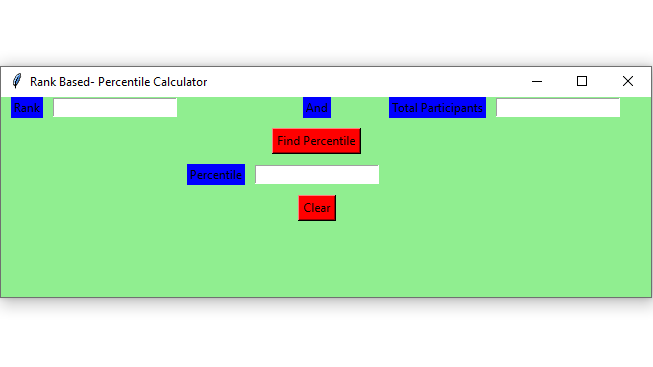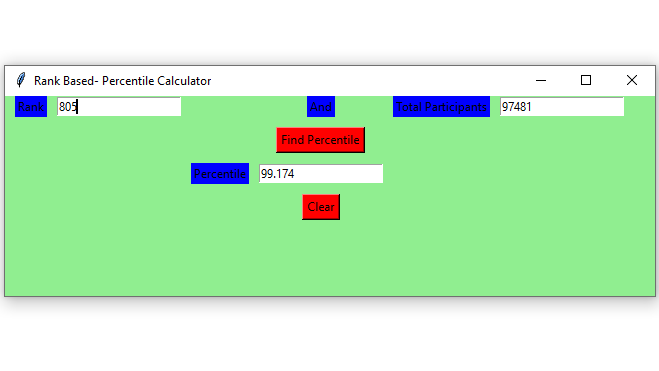# Rank Based Percentile Gui Calculator using Tkinter

Python offers multiple options for developing a GUI (Graphical User Interface). Out of all the GUI methods, Tkinter is the most commonly used method. In this article, we will learn how to create Rank Based – Percentile Gui Calculator application using Tkinter, with a step-by-step guide.

To create a Tkinter:

• Importing the module – tkinter
• Create the main window (container)
• Add any number of widgets to the main window.
• Apply the event Trigger on the widgets.

Let’s create a GUI based simple Rank Based – Percentile Gui Calculator application :

Below is the implementation :

 `# import all functions from the tkinter     ` `from` `tkinter ``import` `*` ` `  `  `  `# Function to calculate the percentile   ` `def` `getPercentile() : ` `  `  `    ``# take a value from the respective entry boxes  ` `    ``# get method returns current text as string  ` `    ``students``=` `int``(total_participantField.get()) ` `      `  `    ``rank ``=` `int``(rankField.get()) ` `  `  `    ``# variable to store the result upto 3 ` `    ``# decimal points   ` `    ``result ``=` `round``((students ``-` `rank) ``/` `students ``*` `100``,``3``); ` `  `  `    ``# insert method inserting the    ` `    ``# value in the text entry box.  ` `    ``percentileField.insert(``10``, ``str``(result))  ` `    `  `      `  `# Function for clearing the    ` `# contents of all text entry boxes  ` `def` `Clear(): ` `      `  `    ``# deleting the content from the entry box  ` `    ``rankField.delete(``0``, END) ` `      `  `    ``total_participantField.delete(``0``, END) ` `      `  `    ``percentileField.delete(``0``, END) ` `      `  `  `  `# Driver Code ` `if` `__name__ ``=``=` `"__main__"` `: ` `  `  `    ``# Create a GUI window  ` `    ``gui ``=` `Tk()  ` `    `  `    ``# Set the background colour of GUI window    ` `    ``gui.configure(background ``=` `"light green"``)  ` `    `  `    ``# set the name of tkinter GUI window   ` `    ``gui.title(``"Rank Based- Percentile Calculator"``)  ` `    `  `     ``# Set the configuration of GUI window  ` `    ``gui.geometry(``"650x200"``)  ` `  `  `    ``# Create a Rank: label   ` `    ``rank ``=` `Label(gui, text ``=` `"Rank"``, bg ``=` `"blue"``) ` `  `  `    ``# Create a And: label   ` `    ``andl ``=` `Label(gui, text ``=` `"And"``, bg ``=` `"blue"``) ` `    `  `    ``# Create a Total Participants : label  ` `    ``total_participant ``=` `Label(gui,  ` `                              ``text ``=` `"Total Participants"``,  ` `                              ``bg ``=` `"blue"``)  ` `  `  `    ``# Create a Find Percentile Button and  ` `    ``# attached to getPercentile function  ` `    ``find ``=` `Button(gui, text ``=` `"Find Percentile"``,  ` `                  ``fg ``=` `"Black"``, bg ``=` `"Red"``, ` `                  ``command ``=` `getPercentile) ` `      `  `    ``# Create a Percentile : label   ` `    ``percentile ``=` `Label(gui, text ``=` `"Percentile"``, bg ``=` `"blue"``)  ` `  `  `    ``# Create a Clear Button and attached  ` `    ``# to Clear function  ` `    ``clear ``=` `Button(gui, text ``=` `"Clear"``, ` `                   ``fg ``=` `"Black"``, bg ``=` `"Red"``, ` `                   ``command ``=` `Clear)  ` `  `  `    ``# grid method is used for placing    ` `    ``# the widgets at respective positions    ` `    ``# in table like structure . ` `  `  `    ``# padx attributed provide x-axis margin   ` `    ``# from the root window to the widget.  ` `    ``rank.grid(row ``=` `1``, column ``=` `1``,padx ``=` `10``) ` `  `  `    ``andl.grid(row ``=` `1``, column ``=` `4``) ` `                   `  `    ``total_participant.grid(row ``=` `1``, column ``=` `6``, padx ``=` `10``) ` `  `  `    ``# pady attributed provide y-axis  ` `    ``# margin from the widget.     ` `    ``find.grid(row ``=` `3``, column ``=` `4``,pady ``=` `10``) ` `      `  `    ``percentile.grid(row ``=` `4``, column ``=` `3``,padx ``=` `10``) ` `      `  `    ``clear.grid(row ``=` `5``, column ``=` `4``,pady ``=` `10``) ` `  `  `    ``# Create a text entry box for filling or ` `    ``# typing the information.    ` `    ``rankField ``=` `Entry(gui) ` `      `  `    ``total_participantField ``=` `Entry(gui) ` `      `  `    ``percentileField ``=` `Entry(gui) ` `  `  `    ``# grid method is used for placing    ` `    ``# the widgets at respective positions    ` `    ``# in table like structure .  ` `    ``rankField.grid(row ``=` `1``, column ``=` `2``) ` `      `  `    ``total_participantField.grid(row ``=` `1``, column ``=` `7``) ` `      `  `    ``percentileField.grid(row ``=` `4``, column ``=` `4``) ` `      `  `    ``# Start the GUI  ` `    ``gui.mainloop()  `

Output :My Personal Notes arrow_drop_upCheck out this Author's contributed articles.

If you like GeeksforGeeks and would like to contribute, you can also write an article using contribute.geeksforgeeks.org or mail your article to contribute@geeksforgeeks.org. See your article appearing on the GeeksforGeeks main page and help other Geeks.

Please Improve this article if you find anything incorrect by clicking on the "Improve Article" button below.

Article Tags :

Be the First to upvote.

Please write to us at contribute@geeksforgeeks.org to report any issue with the above content.